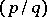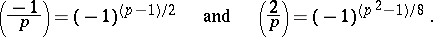# Gauss reciprocity law

(diff) ← Older revision | Latest revision (diff) | Newer revision → (diff)

A relation connecting the values of the Legendre symbols (cf. Legendre symbol)andfor different odd prime numbersand(cf. Quadratic reciprocity law). In addition to the principal reciprocity law of Gauss for quadratic residues, which may be expressed as the relationthere are two more additions to this law, viz.:The reciprocity law for quadratic residues was first stated in 1772 by L. Euler. A. Legendre in 1785 formulated the law in modern form and proved a part of it. C.F. Gauss in 1801 was the first to give a complete proof of the law ; he also gave no less than eight different proofs of the reciprocity law, based on various principles, during his lifetime.

Attempts to establish the reciprocity law for cubic and biquadratic residues led Gauss to introduce the ring of complex integers.

How to Cite This Entry:
Gauss reciprocity law. Encyclopedia of Mathematics. URL: http://encyclopediaofmath.org/index.php?title=Gauss_reciprocity_law&oldid=13461
This article was adapted from an original article by S.A. Stepanov (originator), which appeared in Encyclopedia of Mathematics - ISBN 1402006098. See original article